# Optical properties of materials¶

Solcore has several ways of accessing the optical properties of materials: databases and parametric dielectric functions.

## Dielectric constants models and Adachi parametrization¶

Understanding the optical response of both established and novel materials is crucial to effective solar cell design. To efficiently model the complex dielectric function of a material Solcore incorporates an optical constant calculator based on the well-known Critical-Point Parabolic-Band (CPPB) formalism popularised by Adachi (, , ). In this model, contributions to :math:epsilon_2(omega) from critical points in the Brillouin Zone at which the probability for optical transitions is large (van Hove singularities) are considered. The transition probability for such transitions is proportional to the joint density of states (JDOS)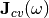, which describes the number of available electronic states between the valence and conduction bands at given photon energy. The imaginary part of the complex dielectric function is related to the JDOS by:Where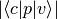is the momentum matrix element for transitions from the valence band (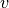) to the conduction band (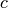). Critical point transitions are considered at the following points of symmetry in the band structure: :math:E_0 corresponds to the optical transition at the :math:Gamma point and :math:E_0 + Delta_0 to the transition from the spin-orbit split off band to the conduction band at the :math:Gamma point. :math:E_1 and :math:E_1 + Delta_1 denote the transitions from the valence heavy-hole (HH) band and the valence light-hole (LH) band respectively to the conduction band at the L point. The :math:E’_0 triplet and :math:E_2 transitions occur at higher energies, between the HH band and the split conduction bands at the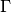point as well as across the wide gap X valley. The model also includes contributions from the lowest energy indirect band-gap transition and the exciton absorption at the :math:E_0 critical point. The contributions listed above are summed to compute the overall value of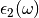. The real and imaginary components of the overall complex dielectric function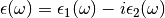are then related via the Kramers-Kronig relations;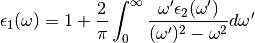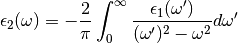The CPPB model included with Solcore also incorporates a modification to the critical point broadening present in Adachi’s description, which is shown to produce a poor fit to experimental data in the vicinity of the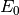and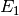critical points (). To give a more accurate description of the broadening of the optical dielectric function, Kim et al. proposed that a frequency-dependent damping parameter be used to replace the damping constant given by Adachi at each critical point (, );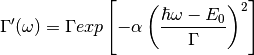Whereis the damping constant used by Adachi and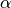describes the shape of the lineshape broadening with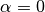producing purely Lorentzian character and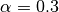producing a good approximation to Gaussian broadening.

The Solcore module absorption_calculator contains the CPPB model within the Custom_CPPB class. The class offers a flexible way to build up the optical constant model by adding individual critical point contributions through the Oscillator structure type within Solcore. In addition to the oscillator functions described by Adachi the Custom_CPPB class also provides additional oscilator models and the Sellmeier equation for describing the real part of the dielectric function for non-absorbing materials ().

Description of functions in this module

This module contains a collection of mathematical models to calculate the dielectric constant of a material. The modelling is largely based in the equations and naming used by:

1. Woollam, Guide to using WVASE: Spectroscopic Ellipsometry Data Acquisition and Analysis Software. 2012, pp. 1–696.
class solcore.absorption_calculator.dielectric_constant_models.Poles(A=0.0, Ec=5.0)[source]

Basic oscillator model for fitting ellipsometry and RAT data. It a pole without broadening and therefore can only represent non-absorbing materials. Usually, the central energy must lie outside the spectral range of interest.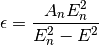with:

• An = the amplitud of the oscillator (dimensionless)
• En = the central energy of the pole (in eV)

It is equivalent to Pol.2 in WVASE

name = 'poles'
var = 2
dielectric(x)[source]

Returns the dielectric function as modelled by this class at the given x input values (a single value or an array of values.

Parameters: x – Spectral position in nm The dielectric constant at this spectral position
class solcore.absorption_calculator.dielectric_constant_models.Lorentz(An=0.0, En=2.0, Brn=0.1)[source]

Classic Lorentz oscillator involving a central energy and a broadening responsible for the absorption.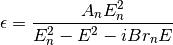with:

• An = the amplitud of the oscillator (dimensionless)
• Brn = the broadening (eV)
• En = the central energy (eV)

It is equivalent to Lor.2 in WVASE.

name = 'lorentz'
var = 3
dielectric(x)[source]

Returns the dielectric function as modelled by this class at the given x input values (a single value or an array of values.

Parameters: x – Spectral position in nm The dielectric constant at this spectral position
class solcore.absorption_calculator.dielectric_constant_models.Gauss(A=0.0, Ec=2.0, Br=0.1)[source]

Gauss oscillator involving a central energy and a broadening responsible for the absorption. It is defined as: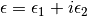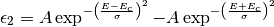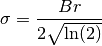Epsilon_1 is calculated using the Kramers-Kronig relationships

with:

• A = the amplitud of the oscillator (dimensionless)
• Br = the broadening (eV)
• Ec = the central energy (eV)

It is equivalent to Gau.0 in WVASE.

name = 'gauss'
var = 3
dielectric(x)[source]

Returns the dielectric function as modelled by this class at the given x input values (a single value or an array of values.

Parameters: x – Spectral position in nm The dielectric constant at this spectral position
class solcore.absorption_calculator.dielectric_constant_models.Drude(An=0.0, Brn=0.1)[source]

The classic Drude oscillator describes free carrier effects on the dielectric response. Its form is a Lorentz oscillator with zero center energy.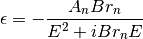with:

• An = the amplitud of the oscillator (eV)
• Brn = the broadening (eV)

It is equivalent to Drd.0 in WVASE.

name = 'drude'
var = 2
dielectric(x)[source]

Returns the dielectric function as modelled by this class at the given x input values (a single value or an array of values.

Parameters: x – Spectral position in nm The dielectric constant at this spectral position
class solcore.absorption_calculator.dielectric_constant_models.Cauchy(An=0.0, Bn=0.0, Cn=0.0, Ak=0.0, Bk=0.0, Ck=0)[source]

Cauchy oscillator model for fitting ellipsometry data. It is generally used to fit non absorbing materials, although an Urbach tail is included to indicate the onset of absorption.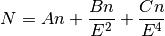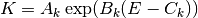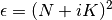with:

• An, Bn and Cn = the Cauchy coeficients (dimensionless, µm-2 and µm-4, respectively)
• Ak = the amplitud of the Urbach tail (dimensionless)
• Bk = the multiplicative factor of the Urbach tail (eV-1)
• Ck = the energy offset of the Urbach tail (eV) - it is user selected, but redundant with Ak.

with Ak.

It is equivalent to Chy.0 in WVASE.

name = 'cauchy'
var = 5
dielectric(x)[source]

Returns the dielectric function as modelled by this class at the given x input values (a single value or an array of values.

Parameters: x – Spectral position in nm The dielectric constant at this spectral position
class solcore.absorption_calculator.dielectric_constant_models.PolySegment(energy, e2)[source]

The PolySegment model aims to approximate the dielectric function with a finite number of linear segments. It is similar to a point-by-point fitting but likely using much less free variables. The segments are created for Epsilon_2 and Epsilon_1 is calculated using the Kramers-Kronig relationships.

It allows for a maximum freedom for fitting data while reducing noise, but does not provide any physically relevant information.

The input parameters are the energies of the endpoints of the segments and the corresponding Epsilon_2 values.

name = 'polysegment'
dielectric(x)[source]

Returns the dielectric function as modelled by this class at the given x input values (a single value or an array of values.

Parameters: x – Spectral position in nm The dielectric constant at this spectral position
class solcore.absorption_calculator.dielectric_constant_models.Oscillator(oscillator_type, material_parameters=None, **kwargs)[source]
class solcore.absorption_calculator.dielectric_constant_models.DielectricConstantModel(e_inf=1.0, oscillators=())[source]

This class groups together several models for the dielectric constant applicable to a given layer. Based on all of them, it calculates the dielectric constant and the complex refractive index at any energy. It requires as input an offset, offen refered as epsilon_infinity.

add_oscillator(name, **kwargs)[source]

Adds an oscillator to the dielectric constant model.

Parameters: name – The name of the oscillator kwargs – The arguments needed to create that oscillator.
remove_oscillator(idx)[source]

Removes an oscillator from the model.

Parameters: idx – The number of the oscillator (starting in 1) None
dielectric_constants(x)[source]

Returns the complex dielectric constant in the form e1 + ie2

Parameters: x – The spectral possition in nm (it can be an array) The complex dielectric constant.
n_and_k(x)[source]

Returns the complex refractive index in the form n + ik

Parameters: x – The spectral possition in nm (it can be an array) The complex refractive index.
solcore.absorption_calculator.adachi_alpha.create_adachi_alpha(material, Esteps=(1.42, 6, 3000), T=300, wl=None)[source]

Calculates the n, k and absorption coefficient of a material using Adachi’s formalism of critical points.

Parameters: material – A solcore material Esteps – (1.42, 6, 3000) A tuple with the start, end and step energies in which calculating the optical data T – Temeprature in kelvin wl – (None) Optional array indicating the wavelengths in which calculating the data A tuple containing 4 arrays: (Energy, n, k, alpha)

## SOPRA database¶

n order to calculate and model the optical response of potential solar cell architectures and material systems, access to a library of accurate optical constant data is essential. Therefore, Solcore incorporates a resource of freely available optical constant data measured by Sopra S. A. and provided by Software Spectra Inc. The refractive index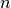and extinction coefficient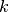are provided for over 200 materials, including many III-V, II-VI and group IV compounds in addition to a range of common metals, glasses and dielectrics.

Any material within the Sopra S.A. optical constant database can be used with the “material” function, but they will have only the optical parametersand. In the case of materials that are in both databases, the keyword “sopra” will need to be set to “True” when creating the material. Once a material is loaded its,and absorption coefficient data is returned by calling the appropriate method, for example SiO2.n(wavelength) and SiO2.k(wavelength). For certain materials in the database, the optical constants are provided for a range of alloy compositions. In these cases, any desired composition within the range can be specified and the interpolatedanddata is returned.

This module gives access to the vast library of optical constant data, made freely available by the SOPRA-SA optoelectronics company founded in 1948. For further detail on the data and SOPRA-SA see the legacy website: http://www.sspectra.com/sopra.html

class solcore.absorption_calculator.sopra_db.sopra_database(Material)[source]

Import the SOPRA_DB module from the solcore.material_system package and get started by selecting a material from the extensive list that SOPRA-SA compiled;

>>> GaAs = sopra_database('GaAs')


Once imported a number of useful methods can be called to return n, k and alpha data for the desired material.

static material_list()[source]

SOPRA_DB.material_list() :: Loads a list (.pdf file) of all available SOPRA materials.

load_n(Lambda=None)[source]

SOPRA_DB.load_n(Lambda) :: Load refractive index (n) data of the requested material. Optional argument Lambda allows user to specify a custom wavelength range. data will be interpolated into

this range before output.

Returns: Tuple of (Wavelength, n)

load_k(Lambda=None)[source]

SOPRA_DB.load_k(Lambda) :: Load refractive index (n) data of the requested material. Optional argument Lambda allows user to specify a custom wavelength range. data will be interpolated into

this range before output.

Returns: Tuple of (Wavelength, k)

load_alpha(Lambda=None)[source]

SOPRA_DB.load_alpha(Lambda) :: Load refractive index (n) data of the requested material. Optional argument Lambda allows user to specify a custom wavelength range. data will be interpolated into

this range before output.

Returns: Tuple of (Wavelength, alpha)

load_temperature(Lambda, T=300)[source]
SOPRA_DB.load_temperature(T, Lambda) :: Loads n and k data for a set of materials with temperature dependent
data sets

Optional argument T defaults to 300K Required argument Lambda specifies a wavelength range and the data is interpolated to fit. This is a

required argument here as not all data sets in a group are the same length (will be fixed in a subsequent update).

Returns: Tuple of (Wavelength, n, k)

load_composition(Lambda, **kwargs)[source]

SOPRA_DB.load_temperature(T, Lambda) :: Loads n and k data for a set of materials with varying composition. Required argument Lambda specifies a wavelength range and the data is interpolated to fit. This is a

required argument here as not all data sets in a group are the same length (will be fixed in a subsequent update).

Keyword argument :: Specify the factional material and fraction of the desired alloy.

Returns: Tuple of (Wavelength, n, k)

exception solcore.absorption_calculator.sopra_db.SOPRAError(message)[source]

## Manually changing optical constants of a material¶

If you would like to define a material with optical constant data from a file, you can do this by telling Solcore the path to the optical constant data, e.g.:

this_dir = os.path.split(__file__)

SiGeSn = material('Ge')(T=T, electron_mobility=0.05, hole_mobility=3.4e-3)

SiGeSn.n_path = this_dir + '/SiGeSn_n.txt'
SiGeSn.k_path = this_dir + '/SiGeSn_k.txt'


In this case, we have defined a material which is like the built-in Solcore germanium material, but with new data for the refractive index and extinction coefficient from the files SiGeSn_n.txt and SiGeSn_k.txt, respectively, which are in the same folder as the Python script. The format of these files is tab-separated, with the first column being wavelength (in nm) and the second column n or k.